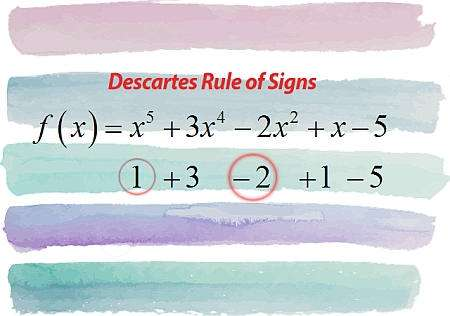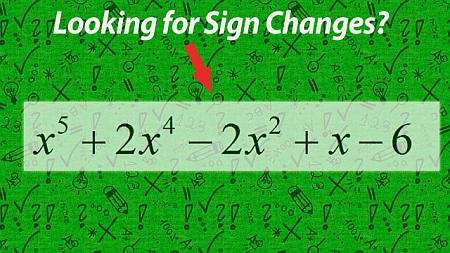# Polynomial Equations

Instructions: Use calculator to solve a polynomial equation that you provide, showing all the steps. Please type the polynomial equation you want to solve in the form below .Enter the polynomial equation you want to solve (Ex: x^3 = x^2, etc.)

Use this calculator to help you solve polynomial equations, showing all the steps of the process. The equation you provide can have polynomial terms on the left and right of the equation.

For example, you can provide an equation like 3x^3 - 2x = 1 + x, which could be derived from trying to find the intersection of the graphs of a cubic and a linear function. Any polynomial equation will do, with integer or fraction coefficients, or any valid numeric expression.

Once a polynomial equation is typed into the form box, you need to click on "Calculate", that will show all the steps of the process and solutions.

One disclaimer, not all polynomial equations can be solved with basic tools. There is no systematic formula to deal with polynomial equation of degree 5 or higher. Also, we deal with the added difficulty that the solutions to a polynomial equation can be complex numbers.## What is a polynomial Equation

A polynomial equation, in simple terms, is an equation in which both sides contain polynomials. Mathematically, a polynomial equation is of the form:

$\displaystyle p(x) = q(x)$

where $$p(x)$$ and $$q(x)$$ are polynomials. For example $$3x+1 = x^2-2$$ is a polynomial equation, but $$\sin(3x+1) = x^2-2$$ is not.

## What are the steps for adding fractions?

• Step 1: Identify the equation you want to work with, indicating clearly the terms on the left and right side, and make sure they are polynomials
• Step 2: Simplify each side as much as possible. Pass all the terms on one of the sides to the other (if both sides have terms)
• Step 3: Now you have a polynomial equation that is set to be equal to zero, so we need to find the roots of the polynomial
• Step 4: We try with possible rational roots, polynomial division for reduction and quadratic formula, as shown in the polynomial zero calculator to find the solutions, if possible

You will find that solving polynomial equations, as finding roots of a polynomial is far from trivial for all cases. Surely, some specific examples can be very simple, but when the exponent of the polynomials involved is large, the process can be very difficult or simply impossible.

## Are Quadratic Equations also Polynomial Equations?

Yes indeed! A quadratic equation is an equation with a polynomial of degree 2 on the left side, and 0 (which is a polynomial too) on the right side, so it fits on the definition.

Indeed, quadratic equations are about the best we can solve with simple tools. Though there are formulas for cubic and quartic equations, there is no general formula for degree 5 or higher. So then we rely on computers to find numerical approximations often times.

Also, not only the exponent of the polynomial can make an equation hard to solve, also cumbersome polynomial coefficients can certainly make things more difficult.

## How are graph of polynomials related to polynomial equations?

There are different ways of seeing it, but one way is to notice that by trying to find the intersection of different polynomials, we are indeed solving a polynomial equation. So there are tightly related problems.### Example: Solving polynomial equations

Calculate the following polynomial equation: $$x^2 = x^3$$

Solution: We have to solve $$x^2 = x^3$$, so we pass $$x^3$$ to the other side, so we get

$x^2 - x^3 = 0$

$x^2(1 - x) = 0$

So then there are two solutions: $$x_1 = 0$$ (which has multiplicity 2), and $$x_2 = 1$$.

### Example: Solving polynomial Equations

What are the solutions of the following equation: $$\frac{2}{3} x^2 + \frac{5}{4} x = \frac{1}{3} x^2 - \frac{5}{6}$$

Solution:We need to solve the following equation:

$\displaystyle \frac{2}{3}x^2+\frac{5}{4}x=\frac{1}{3}x^2-\frac{5}{6}$

Initial Step: In this case, we first need to simplify the given equation $$\displaystyle \frac{2}{3}x^2+\frac{5}{4}x=\frac{1}{3}x^2-\frac{5}{6}$$, by putting all the terms on one side of the equation, so we get:

$$\displaystyle \frac{2}{3}x^2+\frac{5}{4}x-\left(\frac{1}{3}x^2-\frac{5}{6}\right)$$
Removing unnecessary parentheses and multiplying the terms by $$-1$$
$$\displaystyle = \,\,$$
$$\displaystyle \frac{5}{4}x+\frac{2}{3}x^2-\frac{1}{3}x^2+\frac{5}{6}$$
Grouping the terms with $$x^2$$
$$\displaystyle = \,\,$$
$$\displaystyle \frac{5}{4}x+\left(\frac{2}{3}-\frac{1}{3}\right)x^2+\frac{5}{6}$$
Grouping together the fractions and operating the terms that were grouped with $$x^2$$
$$\displaystyle = \,\,$$
$$\displaystyle \frac{5}{4}x+\frac{1}{3}x^2+\frac{5}{6}$$

Hence, after simplifying, we need to solve the following polynomial equation of order $$2$$:

$\displaystyle \frac{1}{3}x^2+\frac{5}{4}x+\frac{5}{6} = 0$

Observe that the degree of the given polynomial is $$\displaystyle deg(p) = 2$$, its leading coefficient is $$\displaystyle a_{2} = \frac{1}{3}$$ and its constant coefficient is $$\displaystyle a_0 = \frac{5}{6}$$.

We need to solve the following given quadratic equation $$\displaystyle \frac{1}{3}x^2+\frac{5}{4}x+\frac{5}{6}=0$$.

For a quadratic equation of the form $$a x^2 + bx + c = 0$$, the roots are computed using the following formula:

$x = \displaystyle \frac{-b \pm \sqrt{b^2-4ac}}{2a}$

In this case, we have that the equation we need to solve is $$\displaystyle \frac{1}{3}x^2+\frac{5}{4}x+\frac{5}{6} = 0$$, which implies that corresponding coefficients are:

$a = \frac{1}{3}$ $b = \frac{5}{4}$ $c = \frac{5}{6}$

First, we will compute the discriminant to assess the nature of the roots. The discriminating is computed as:

$\Delta = b^2 - 4ac = \displaystyle \left( \frac{5}{4}\right)^2 - 4 \cdot \left(\frac{1}{3}\right)\cdot \left(\frac{5}{6}\right) = \frac{65}{144}$

Since in this case we get the discriminant is $$\Delta = \displaystyle \frac{65}{144} > 0$$, which is positive, we know that the equation has two different real roots.

Now, plugging these values into the formula for the roots we get:

$x = \displaystyle \frac{-b \pm \sqrt{b^2-4ac}}{2a} = \displaystyle \frac{-\frac{5}{4} \pm \sqrt{\left(\frac{5}{4}\right)^2-4\left(\frac{1}{3}\right)\left(\frac{5}{6}\right)}}{2\cdot \frac{1}{3}} = \displaystyle \frac{-\frac{5}{4} \pm \sqrt{\frac{65}{144}}}{\frac{2}{3}}$

so then, we find that:

$x_1 = -\frac{\frac{5}{4}}{\frac{2}{3}}-\frac{1}{\frac{2}{3}}\sqrt{\frac{65}{144}}=-\frac{\frac{5}{4}}{\frac{2}{3}}-\frac{1}{\frac{2}{3}}\cdot\frac{1}{12}\sqrt{65}=-\frac{5}{4}\cdot \frac{3}{2}-\frac{\frac{1\cdot 3}{2}\cdot 1}{12}\sqrt{65}=-\frac{15}{8}+1\cdot \left(-\frac{1}{8}\right)\sqrt{65}=-\frac{15}{8}-\frac{1}{8}\sqrt{65}$ $x_2 = -\frac{\frac{5}{4}}{\frac{2}{3}}+\frac{1}{\frac{2}{3}}\sqrt{\frac{65}{144}}=-\frac{\frac{5}{4}}{\frac{2}{3}}+\frac{1}{\frac{2}{3}}\cdot\frac{1}{12}\sqrt{65}=-\frac{15}{8}+1\cdot \frac{1}{8}\sqrt{65}=-\frac{15}{8}+\frac{1}{8}\sqrt{65}$

In this case, the quadratic equation $$\displaystyle \frac{1}{3}x^2+\frac{5}{4}x+\frac{5}{6} = 0$$, has two real roots, so then:

$\displaystyle \frac{1}{3}x^2+\frac{5}{4}x+\frac{5}{6} = \frac{1}{3} \left(x+\frac{1}{8}\sqrt{65}+\frac{15}{8}\right)\left(x-\frac{1}{8}\sqrt{65}+\frac{15}{8}\right)$

so then the original polynomial is factored as $$\displaystyle p(x) = \frac{1}{3}x^2+\frac{5}{4}x+\frac{5}{6} = \frac{1}{3} \left(x+\frac{1}{8}\sqrt{65}+\frac{15}{8}\right)\left(x-\frac{1}{8}\sqrt{65}+\frac{15}{8}\right)$$, which completes the factorization.

Conclusion: The solution to the polynomial equation found using the factorization process are $$-\frac{1}{8}\sqrt{65}-\frac{15}{8}$$ and $$\frac{1}{8}\sqrt{65}-\frac{15}{8}$$ .

## More polynomial calculators

Polynomials equations appear so naturally in Algebra, that they are one of the most important topics in Algebra. When you are looking for the intersection of two parabolas, you will need to solve a polynomial equation, just to mention one situation of many.

Solving polynomial equation is not straightforward, especially for higher polynomial degrees. Indeed, there is as certain possibility that you won't be able to find all the solution of a given equation manually (or any solution for that matter).

The best manual alternative involves grouping all the polynomial terms on one side to reduce it to finding the zeros of a polynomial. Then, we use the quadratic formula when possible, and try to reduce the order of the polynomial by polynomial division and the factor theorem.